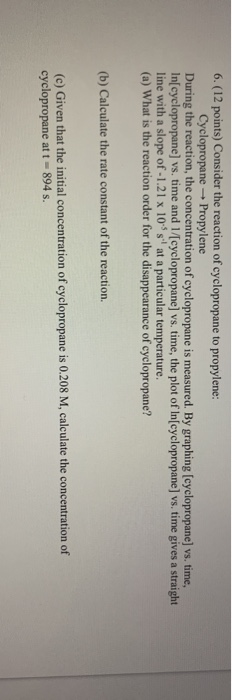### Create an Account

Home / Questions / Consider the reaction of cyclopropane to propylene: Cyclopropane - Propylene During the r...

# Consider the reaction of cyclopropane to propylene: Cyclopropane - Propylene During the reaction, the concentration of cyclopropane is measured. By graphing (cyclopropane) vs. time, Inſcyclopropane)

Consider the reaction of cyclopropane to propylene: Cyclopropane - Propylene During the reaction, the concentration of cyclopropane is measured. By graphing (cyclopropane) vs. time, Inſcyclopropane) vs. time and 1/[cyclopropane) vs. time, the plot of Inſcyclopropane) vs. time gives a straight line with a slope of -1.21 x 10 s' at a particular temperature. (a) What is the reaction order for the disappearance of cyclopropane? (b) Calculate the rate constant of the reaction. (c) Given that the initial concentration of cyclopropane is 0.208 M, calculate the concentration of cyclopropane at t-894 s.Jun 18 2021 View more View LessSubscribe To Get Solution